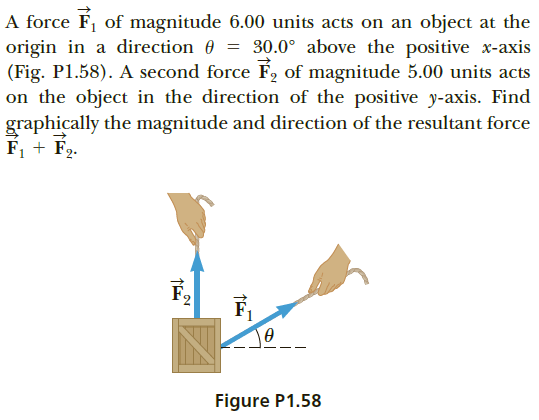# A force F, of magnitude 6.00 units acts on an object at theorigin in a direction 0 = 30.0° above the positive x-axis(Fig. P1.58). A second force É2 of magnitude 5.00 units actson the object in the direction of the positive y-axis. Findgraphically the magnitude and direction of the resultant forceF, + F2.Figure P1.58

Question
13 viewshelp_outlineImage TranscriptioncloseA force F, of magnitude 6.00 units acts on an object at the origin in a direction 0 = 30.0° above the positive x-axis (Fig. P1.58). A second force É2 of magnitude 5.00 units acts on the object in the direction of the positive y-axis. Find graphically the magnitude and direction of the resultant force F, + F2. Figure P1.58 fullscreen
check_circle

Step 1

Two forces and the resultant force vector can be represented graphically as,

Step 2

From the head of the first vector, the second force vector is beginning. The resultant vector starts from the end of the fir...

### Want to see the full answer?

See Solution

#### Want to see this answer and more?

Solutions are written by subject experts who are available 24/7. Questions are typically answered within 1 hour.*

See Solution
*Response times may vary by subject and question.
Tagged in

### Vectors and Scalars